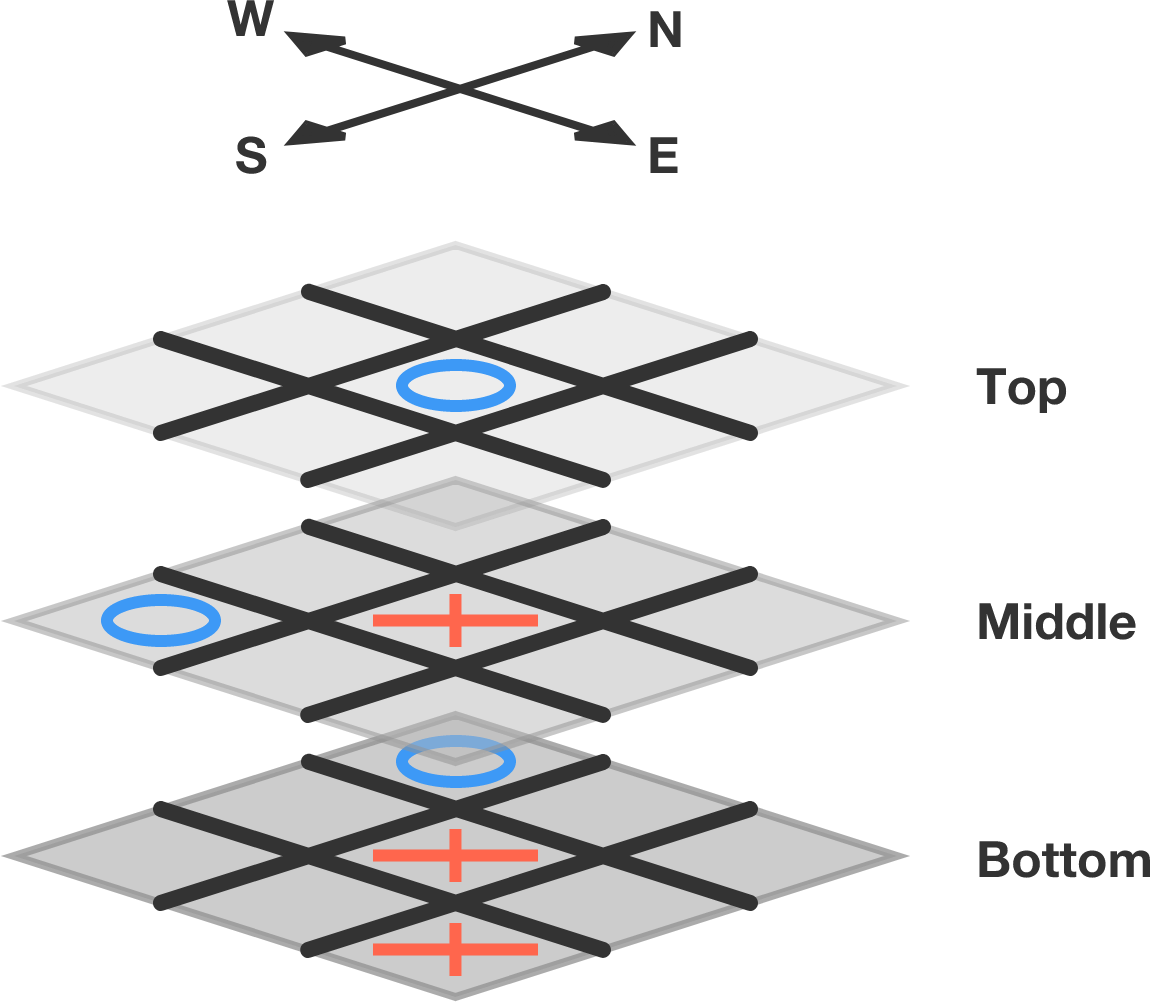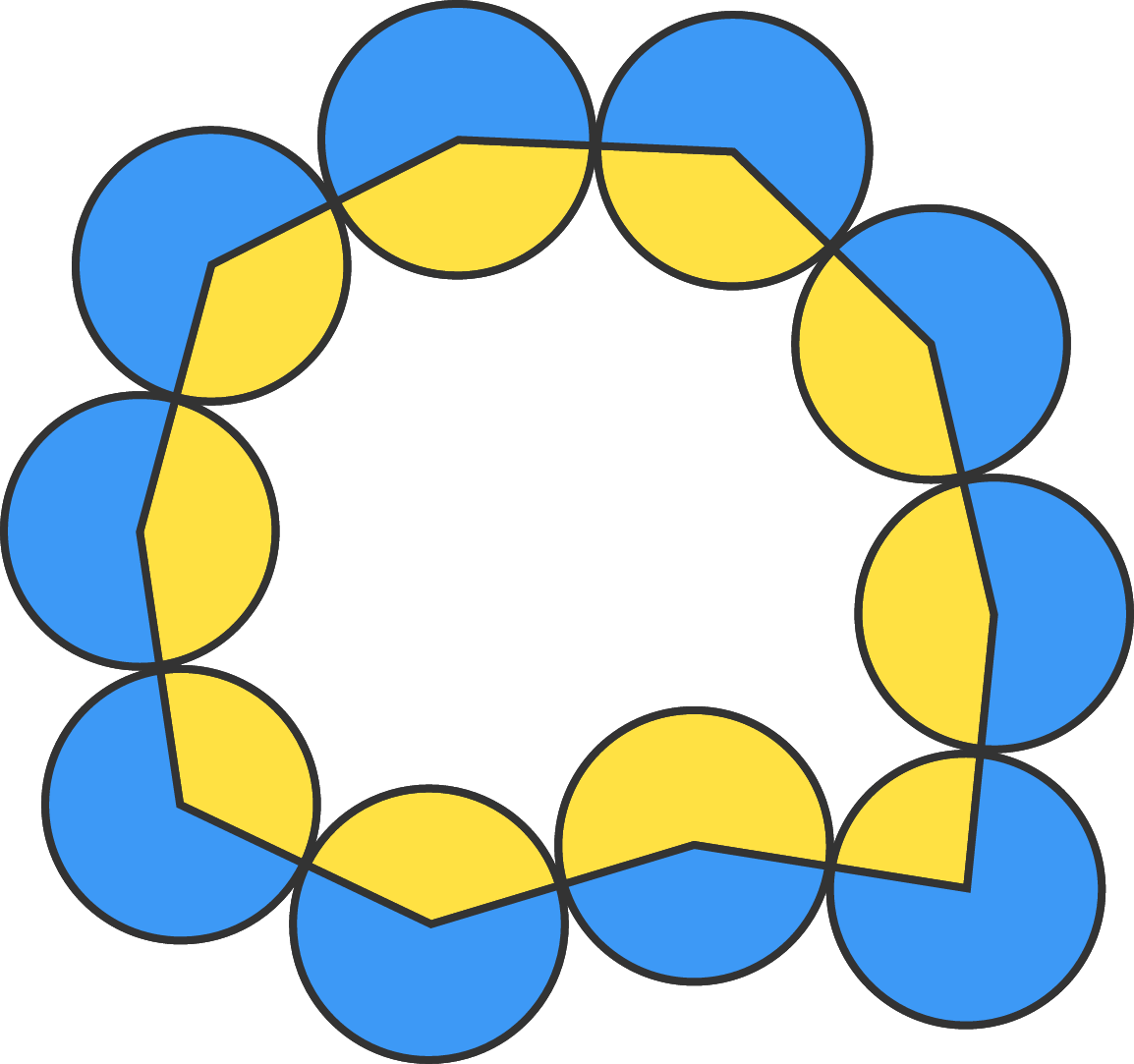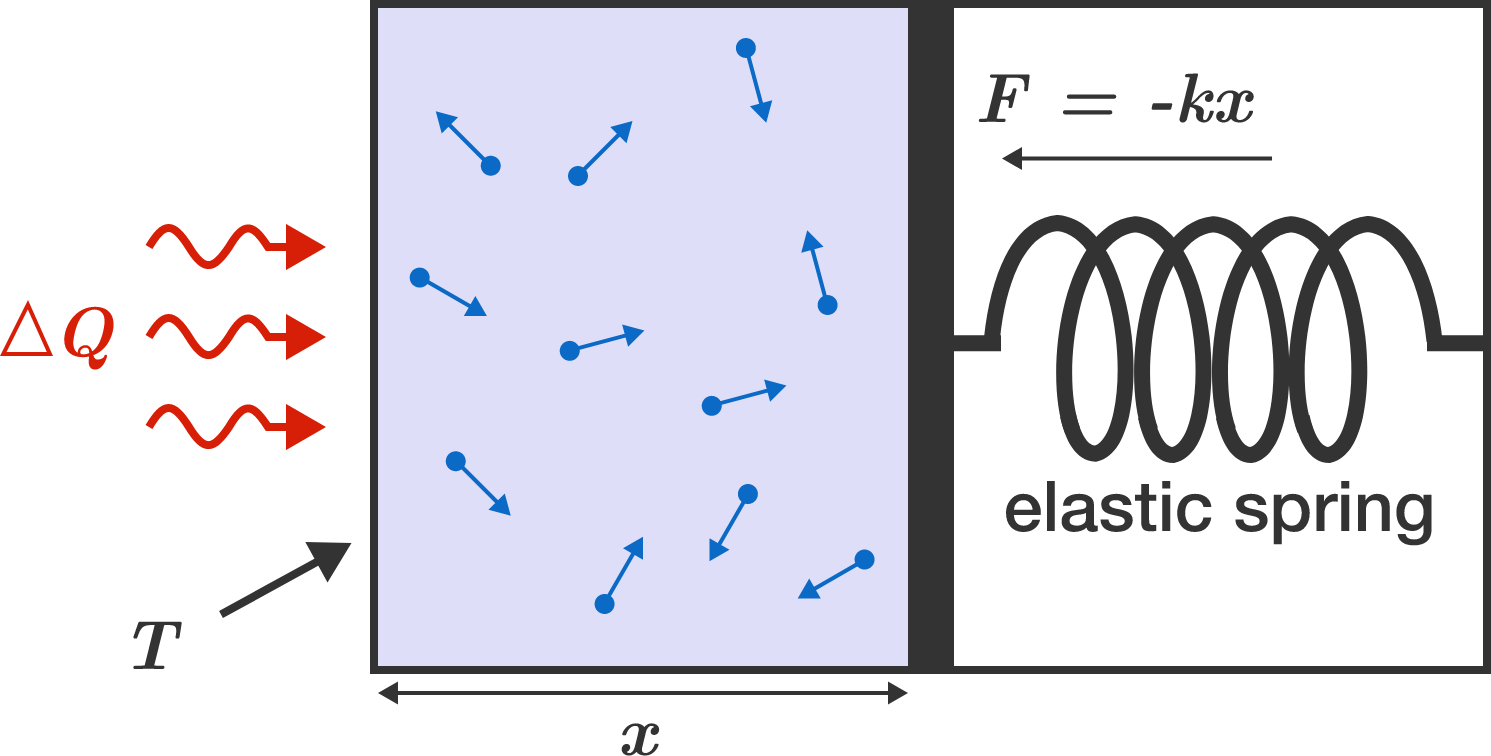# Problems of the Week

Contribute a problem

# 2017-10-16 Intermediate

In a $3 \times 3 \times 3$ tic-tac-toe game, a winning line can go through each layer of the three boards (in addition to the standard winning lines in any 2D plane of a single board). Two players play on this 3-layer board with normal tic-tac-toe rules.

Is it possible to tie in this version of tic-tac-toe?It is $\color{#D61F06}\text{X}$'s turn. $\color{#D61F06}\text{X}$ can win by filling in the Top NW space. The winning line will go through Top NW, Middle Center, and Bottom SE.10 identical circles of radius 2 are externally tangential to one another, as shown.

Connecting the centers of adjacent circles gives a 10-sided polygon, which divides the circles into two areas: blue and yellow. The positive difference between the blue and yellow areas is $k \pi.$

What is $k?$

Does there exist a positive integer $n$ such that $\sqrt{2 \times \sqrt{3 \times \sqrt{\dots \sqrt{(n-1)\times {\sqrt{n}}}}}}\ \ {\large{>3}\hspace{0.3mm}?}$

Is it possible to fill a $2017 \times 2017$ square grid with $2017^2$ distinct positive integers such that the product of the numbers in each row and each column is the same?

Helium gas is enclosed in a gas-tight piston which is held in position by the clamping force of an elastic spring. In mechanical equilibrium, the gas pressure $p = -\frac FA$ corresponds to the tensioning force $F$ of the spring divided by the area $A$. The gas is then brought to a temperature of $T = 600 \,\text{K}$ by rapid heating from room temperature $T = 300 \,\text{K}$.

What is the final temperature of the gas (in units of Kelvin) after setting a new mechanical balance with the spring?Details and Assumptions:

• The volume of the gas is $V = Ax,$ where $A$ is the cross-sectional area and $x$ is the piston position.
• The mechanical force of the spring equals $F = - k x,$ where $k$ is the spring constant.
• The piston does not move during the heating from $300 \,\text{K}$ to $600 \,\text{K}$.
• Helium can be treated as an ideal gas.
• Energy is transferred only by mechanical work. The piston is perfectly thermally insulated.
×